# Practical File Class 12 IP 2022-23

Data Handling

## Program 1: Create a panda’s series from a dictionary of values and a ndarray

```'''Python program to create a panda’s series
from a dictionary of values and a ndarray'''

import pandas as pd

import numpy as np

s=pd.Series(np.array([2,4,5,7,9,8,9]))

print(s)
```

Output:

```0 2
1 4
2 5
3 7
4 9
5 8
6 9
dtype: int32
>>>```
```# import panda lib as pd
import pandas as pd

# creating a dictionary

dictionary={'A':10, 'B':20, 'C':30}

#creating a series

series=pd.Series(dictionary)

print(series)```

Output:

```A 10
B 20
C 30
dtype: int64
>>>```

## Program 2:Given a Series, print all the elements that are above the 75th percentile.

```# import panda lib as pd
import pandas as pd
import numpy as np

series=pd.Series(np.array([1,4,6,7,8,9,11]))

print (series)

result=series.quantile(q=0.75)

print()

print ("75 percentile of the series is : ")

print(result)

print()

print ("The Elements above 75 percentile is : ")

print(series[series>result])

```

Output:

```0 1
1 4
2 6
3 7
4 8
5 9
6 11
dtype: int32

75 percentile of the series is :
8.5

The Elements above 75 percentile is :
5 9
6 11
dtype: int32
>>>```

## Program 3: Create a Data Frame quarterly sales where each row contains the item category, item name, and expenditure. Group the rows by the category and print the total expenditure per category.

```# import panda lib as pd
import pandas as pd

dictionary={'item_cat':['Tata', 'Hyundai', 'Maruti', 'Renault'],
'item_name':['Harrier', 'KIA', 'Swift', 'Duster'],
'expenditure':[2000000, 700000, 900000, 1400000]}

quarter_sales=pd.DataFrame(dictionary)

print (quarter_sales)

q_sale=quarter_sales.groupby('item_cat')

print("Result after Filtering DataFrame")

print (q_sale['item_cat','expenditure'].sum())

```

Output:

``` item_cat item_name expenditure
0 Tata Harrier 2000000
1 Hyundai KIA 700000
2 Maruti Swift 900000
3 Renault Duster 1400000
Result after Filtering DataFrame

expenditure
item_cat
Hyundai 700000
Maruti 900000
Renault 1400000
Tata 2000000
>>>```

## Program 4:Create a data frame for examination result and display row labels, column labels data types of each column and the dimensions

```import pandas as pd

dictionary={'class':['I', 'II','III','IV','V','VI','VII','VIII','IX','X','XI','XII', ],
'pass_percentage':[100,100,100,100,100,100,100,100,96,99.10,98,97.7]}

result=pd.DataFrame(dictionary)

print (result)

print (result.dtypes)

print("shape of the dataframe is : ")

print (result.shape)

```

Output:

```class pass_percentage
0 I 100.0
1 II 100.0
2 III 100.0
3 IV 100.0
4 V 100.0
5 VI 100.0
6 VII 100.0
7 VIII 100.0
8 IX 96.0
9 X 99.1
10 XI 98.0
11 XII 97.7
class object
pass_percentage float64
dtype: object
shape of the dataframe is :
(12, 2)
>>>```

## Program 5:Filter out rows based on different criteria such as duplicate rows.

```'''Python program to Filter out rows based
on different criteria such as duplicate rows'''

import pandas as pd

dic={'Name':['Ajay','Banti','Chandrkant','Deepak','Geeta','Harshit'],
'CS_Marks':[80,78,80,92,78,99]}

marks=pd.DataFrame(dic)

print(marks)

#Finding duplicate rows

print("Duplicate Rows : ")

duplicateRow = marks[marks.duplicated('CS_Marks',keep=False)]

print(duplicateRow)
```

Output:

```CS_Marks Name
0 80 Ajay
1 78 Banti
2 80 Chandrkant
3 92 Deepak
4 78 Geeta
5 99 Harshit
Duplicate Rows :
CS_Marks Name
0 80 Ajay
1 78 Banti
2 80 Chandrkant
4 78 Geeta
>>>```

## Program 6:Importing and exporting data between pandas and CSV file

```'''Importing data between pandas and CSV file'''
import pandas as pd

#make data frame

print(df)```

Output:

```ROLL No Student Name Class Marks
0 1 Arnav III 100
1 2 Bikram V 98
2 3 Charu VII 99
3 4 Dhruv VIII 91
4 5 Harshit X 80
>>>```

```'''Exporting data between pandas and CSV file'''

import pandas as pd

dic=[{'Name': 'Amit', 'Class': 12},
{'Name': 'Abhay', 'Class': 10},
{'Name': 'Harshit', 'Class': 9},
{'Name': 'Arnav', 'Class': 11}]

#make data frame

df_1=pd.DataFrame(dic)

# saving the data frame

df_1.to_csv('d:\Dataframe1.csv')```

5.2 Visualization

## Program 7:Given the school result data, analyses the performance of the students on different parameters, e.g subject wise or class wise.

```import matplotlib.pyplot as plt

subject=['English Core','Mathematics', 'Physics', 'Chemistry', 'I.P.']

percentage=[83,95,70, 89, 100]

plt.bar(subject,percentage, align='center', color='green')

plt.xlabel('Subject Name')

plt.ylabel('Student Name')

plt.title('Result Analysis Bar Graph  ')

plt.show()
```

Output: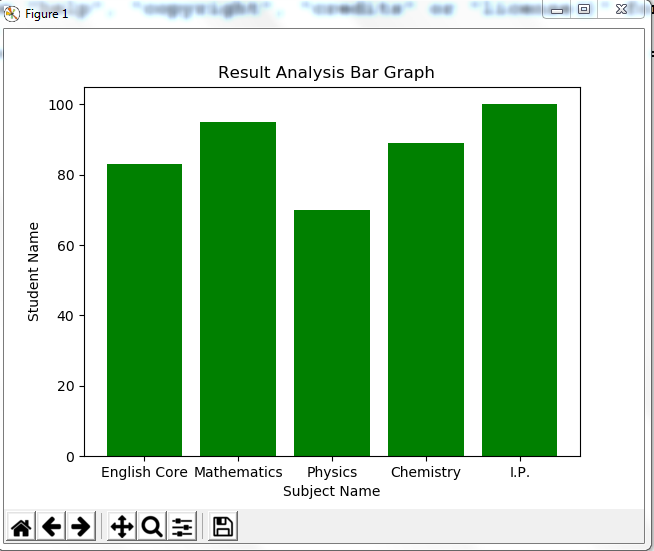## Program 8:For the Data frames created above, analyze, and plot appropriate charts with title and legend.

```import matplotlib.pyplot as plt

import numpy as np

place=['1st','2nd','3rd']

pcm_percentage=[80,90,100]

pcb_percenatge=[90,100,80]

comm_percentage=[100,90,80]

x=np.arange(len(place))

plt.bar(x,pcm_percentage, label='PCM', width=0.25, color='red')

plt.bar(x+.25,pcb_percenatge, label='PCB', width=0.25, color='green')

plt.bar(x+.50,comm_percentage, label='Commerce', width=0.25, color='yellow')

plt.xticks(x,place)

plt.xlabel('Position')

plt.ylabel('Percentage')

plt.title('Result Analysis Bar Graph')

plt.legend()

plt.show()
```

Output: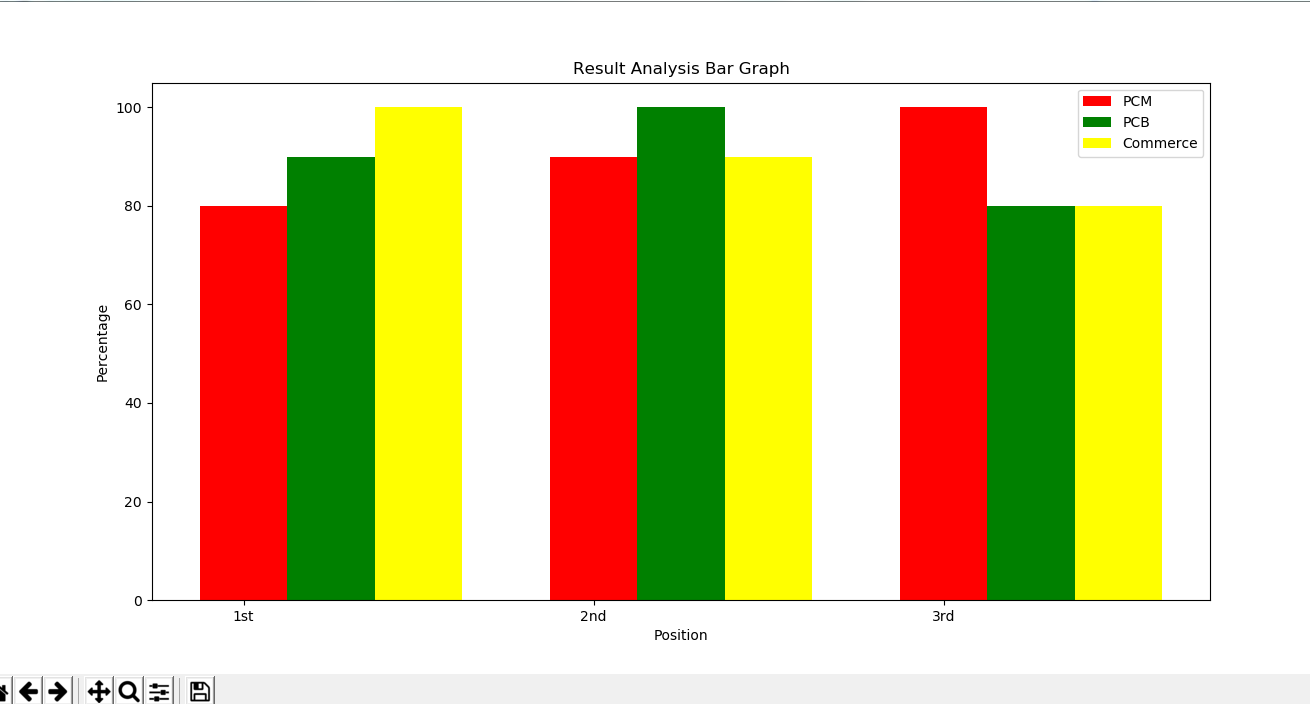## Program 9:Take data of your interest from an open source (e.g. data.gov.in), aggregate and summarize it. Then plot it using different plotting functions of the Matplotlib library.

```import pandas as pd

import matplotlib.pyplot as plt

print(dframe)```

Output: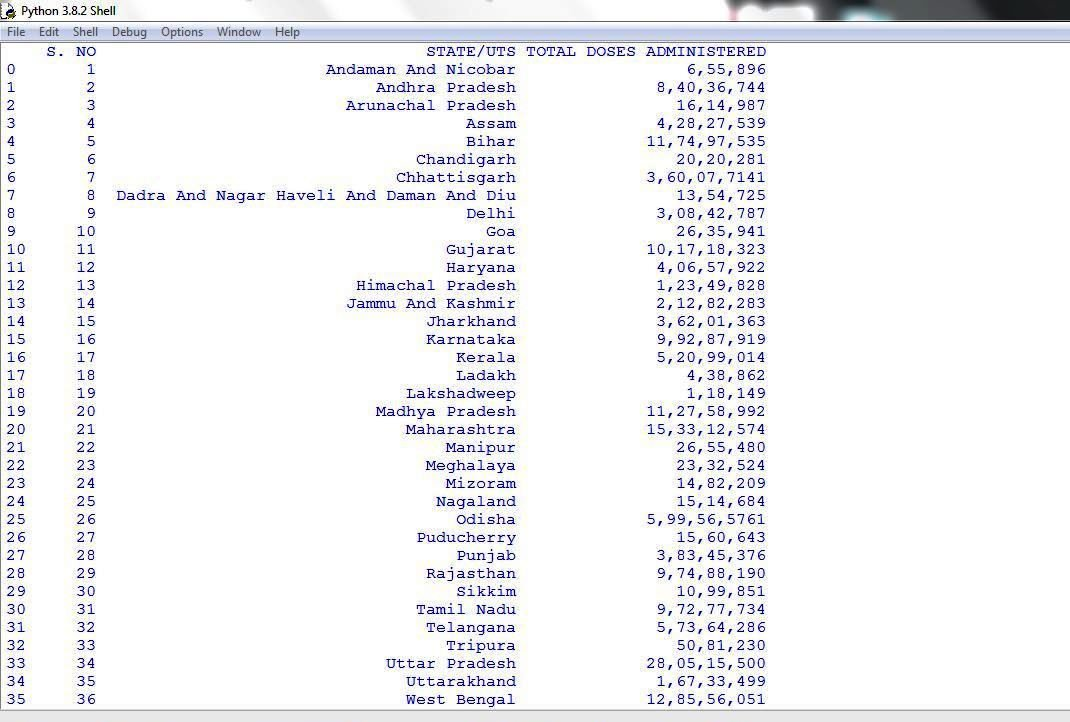```import pandas as pd

import matplotlib.pyplot as plt

colours=['c','b','y','m','r','gold']

exp=[0,0,0,0,0,0.1]

plt.pie(slices, labels=states, colors=colours, startangle=90, explode=exp, shadow=True, autopct='%.1f%%')

plt.legend()

plt.show()
```

Output: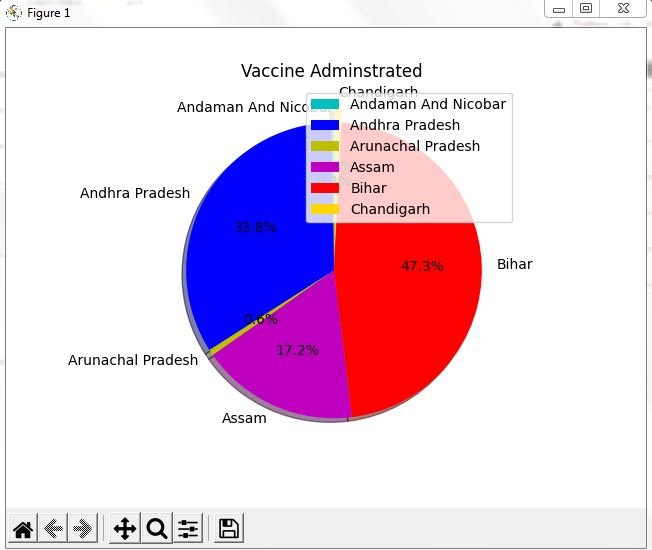## Data Management

### 1. Create a student table with the student id, name, and marks as attributes where the student id is the primary key.

```CREATE TABLE Student
(
Student_ID int Primary Key,
Student_Name  varchar(25),
Marks int
);
```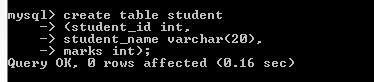### 2. Insert the details of a new student in the above table.

`insert into student values(101, Amit, 87);`### 3. Delete the details of a student in the above table.

`delete from student where Student_ID=101;`

### 4. Use the select command to get the details of the students with marks more than 80.

`select * from student where marks>80;`

### 5. Find the min, max, sum, and average of the marks in a student marks table.

`select max(marks), min(marks), sum(marks) , avg(marks) from student;`

### 6. Write a SQL query to order the (student ID, marks) table in descending order of the marks.

`select * from student order by marks desc;`#### By cbsepython

A complete solution for the students of class 9 to 12 having subject Information Technology (402), Computer Science (083). Explore our website for all the useful content as Topic wise notes, Solved QNA, MCQs, Projects and Quiz related to the latest syllabus.